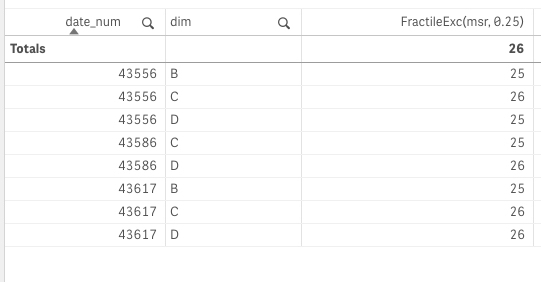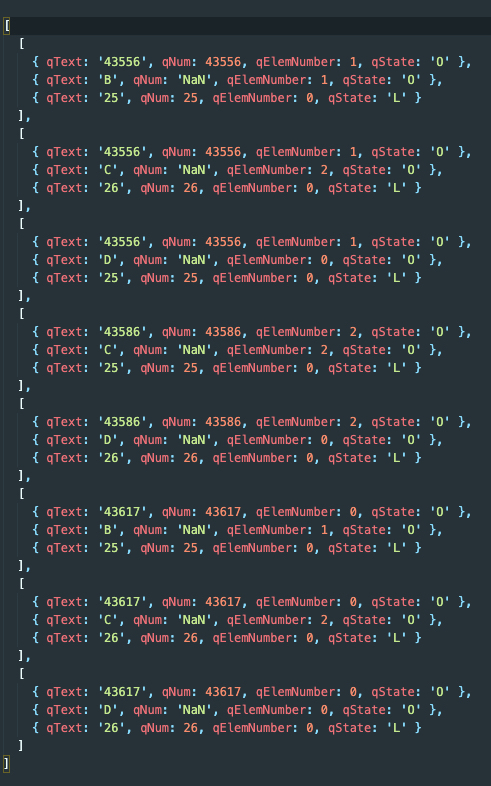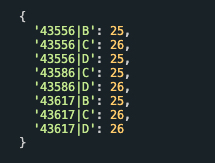Here are a couple ways to take HyperCube data and transform it into something more useable.

## Example

#### Qlik Chart Object

This is a simple chart with two dimensions and one measure.#### HyperCube Data Sample

The hypercube is the object behind a Qlik chart object. Here is how the chart data is returned by the `getHyperCubeData()` API call.#### Row Array

This would create an array for each row, where each value in the list is a column value. This is a simple version of a hypercube data set.#### Object

This would create a single object, where the dimension fields are the key. This is in my opinion the easiest to work with.